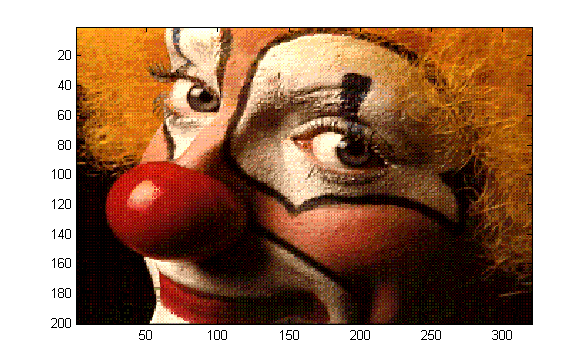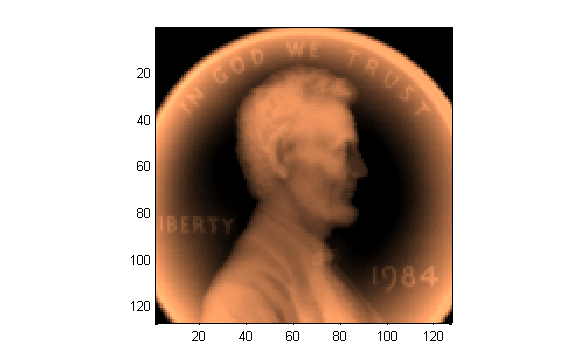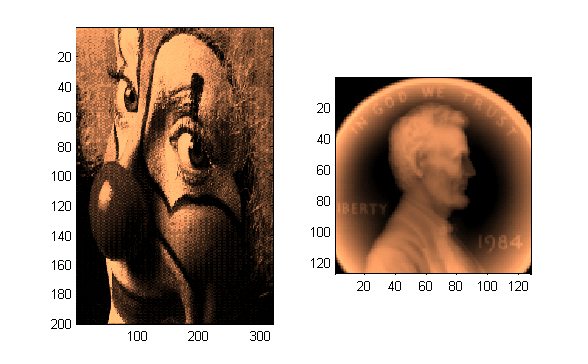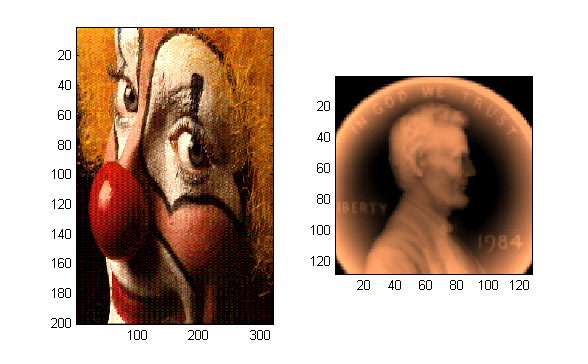# Using multiple colormaps in a single figure

Former "Pickmaster" (and current blogger) Doug Hull suggested that John Iversen's color freezer might be "Pickworthy." I wholeheartedly agree.

Suppose you wanted to display the image of a clown that ships as a MATLAB demo. This snippet would do the trick:

load clown;
image(X);
colormap(map);Similarly, if you wanted to display a penny with a copper colormap, this would work:

load penny.mat
contour(P,15)
colormap(copper)
axis ij square
pcolor(P)
axis ij square
shading flatBut what if you wanted to show the two graphics in one figure window? Colormaps are properties of figures, so it's a bit more complicated to do that than you might think:

figure('color','w')
subplot(1,2,1)
image(X);
colormap(map);
subplot(1,2,2)
contour(P,15)
colormap(copper)
axis ij square
pcolor(P)
axis ij square
shading flatNotice that the second call to colormap affects the image of the clown as well as that of the penny. There are ways to circumvent this behavior. Notably, you could combine the two colormaps into one, and then use different portions of the concatenated colormap for each graphic displayed. Or, if you have the Image Processing Toolbox and are dealing exclusively with images, you can use function subimage to visualize images with different colormaps.

But John's submission makes it considerably easier to combine graphics; just issue a freezeColors command after drawing the clown image, then generate the display of the penny:

figure('color','w')
subplot(1,2,1)
image(X);
colormap(map);
% Here's John's contribution:
freezeColors

subplot(1,2,2)
contour(P,15)
colormap(copper)
axis ij square
pcolor(P)
axis ij square
shading flatI've been meaning to write a similar function for years. Now I don't have to. Thanks, John!

Comments? Leave them here.

Published with MATLAB® 7.8

|